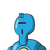# The bill for dinner was $54.38 you left$10.62 for the tip what percentage of the original bill was the tip ? Round to the nearest

The bill for dinner was $54.38 you left$10.62 for the tip what percentage of the original bill was the tip ? Round to the nearest whole percent

### 1 thought on “The bill for dinner was $54.38 you left$10.62 for the tip what percentage of the original bill was the tip ? Round to the nearest”

1.### SOLUTION

GIVEN

The bill for dinner was $54.38 you left$ 10.62 for the tip

TO DETERMINE

The percentage of the original bill was the tip. Round to the nearest whole percent

EVALUATION

Here it is given that the bill for dinner was $54.38 you left$ 10.62 for the tip

So original bill = $54.38 Tip =$ 10.62

Hence the required percentage of the original bill was the tip

$$\displaystyle \sf{ = \frac{10.62}{54.38} \times 100 \: \% }$$

= 19.52%

≈ 20% ( Round to the nearest whole percent )

The required percentage of the original bill was the tip = 20%

━━━━━━━━━━━━━━━━